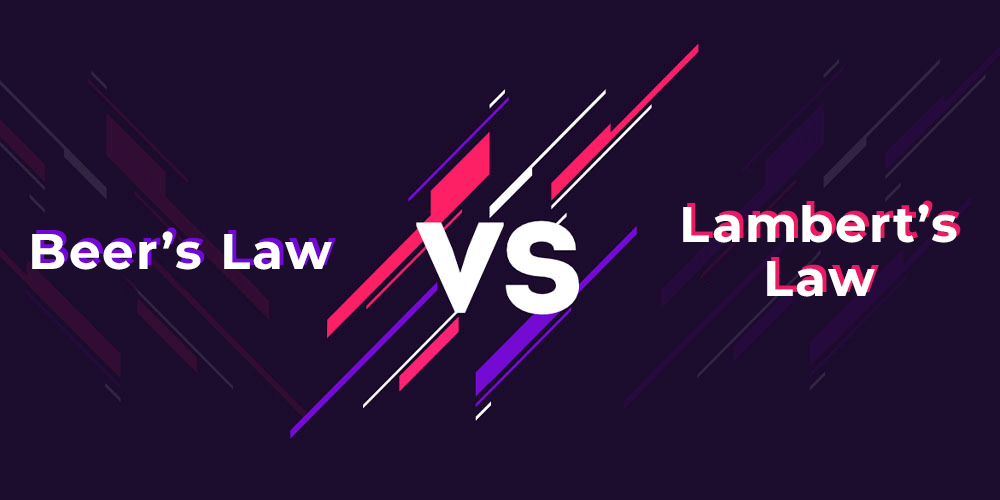GFG App
Open AppBrowser
Continue

# Difference Between Beer’s Law and Lambert’s Law

Beer’s law states that the amount of absorbed light is proportional to the solution concentration, whereas Lambert’s law states that the absorbance and path length are directly linked.

Beer’s law and Lambert’s law are frequently used in combination with the Beer-Lambert law because they can show the connection between absorbance and both the path length of light inside the sample and the sample concentration.### What is Beer’s Law?

According to Beer’s law, the quantity of light absorbed is directly proportional to the solution concentration. This equation is related to the weakening of light to the material properties.

Apart from this, it states that the absorbance of a solution is directly proportional to solvent concentration. Hence, we can utilize this correlation to identify the concentration of a taken sample solution by utilizing a spectrophotometer or colorimeter. Usually, this correlation is helpful in UV-visible absorption spectroscopy. But, this law is valid only for high concentration solutions.

Because so many people were involved in making this law/statement, it’s also known as the Beer-Lambert law, Lambert-Beer law, and Beer-Lambert-Bouguer law. In other words, Beer’s law incorporates several laws proposed by numerous scientists. The following is the equation:

A = ε × l × c

where

• A is the absorbance,
• ε is the molar extinction coefficient,
• l is the length of the path, and
• c is the concentration of the solution.

### What is Lambert’s Law?

Lambert’s law suggests that a sample’s absorbance is proportional to the path length of light passing through it. This law is frequently used in combination with Beer’s law, resulting in the Beer-Lambert law.

This is because, in addition to these separate rules, the Beer-Lambert law is very useful in spectroscopic analysis. Johann Heinrich Lambert was the first to propose Lambert’s law.

### Sample Problems

Problem 1: A student has a sample of Thymine with an absorbance of 0.56 at a wavelength of 360 nm. The Molar absorption coefficient (ε360) is 4250 M−1cm−1. The path length of light is 3 cm. What is the concentration of the sample?

Solution:

Given that,

Absorbance = 0.56

Wavelength = 360nm

Molar absorption coefficient = 4250 M−1cm−1

Path length of light = 3 cm

From the formula , A=εcl

Concentration = A/ εl = 0.56/4250 M−1cm−1 × 3 cm

Therefore, the concentration is 4.39 × 10-5 M.

Problem 2: A scientist has a sample of Cytosine with the molar absorption coefficient of sample at 260 nm is 6000 M−1cm−1. The path length of light is 3.00 cm, then find the absorbance if the concentration of the sample solution is 3.5×10-5M

Solution:

Given that,

Molar absorption coefficient at 260 nm = 6000 M−1cm−1

Path length of light = 3cm

From the formula,

Absorbance. A=εcl

= 6000M−1cm−1 × 3.5×10-5M × 3cm

Therefore, the absorbance is calculated as 0.63.

Problem 3: A researcher has a sample of Sodium chloride with the Molar absorption coefficient at 245 nm is 3000 M−1cm−1 then find the path length if the absorbance is 0.34 and sample concentration is 6×10-4M

Solution:

Given that,

Absorbance = 0.34

Molar absorption coefficient at 245nm = 3000 M−1cm−1

Concentration of sample = 6 × 10-4M

From the formula,

Absorbance A=εcl

l = A/εc

= 0.34/3000 M−1cm−1 × 6 × 10-4

Therefore, the absorbance is calculated as 0.18 cm.

Problem 4: If the concentration of the sample (5 g/liter). The cuvette length is 4 cm and  75% of the beam of light is transmitted. What is the molar absorption coefficient?

Solution:

Given,

Concentration of sample = 5g/l

Path Length = 4 cm

Percentage of light transmitted = 75%

Equation 1 : A= log (I0/ I)

Equation 2 : A= εlc

Combining equations 1 and 2

A= εlc = log 10 (I0/ I)

= log10 (1/0.75)

= log10 1.33 = 0.1238

εlc = ε×4cm×5g/L

= 0.1238

Hence molar absorption coefficient is ε = 0.0062.

My Personal Notes arrow_drop_up